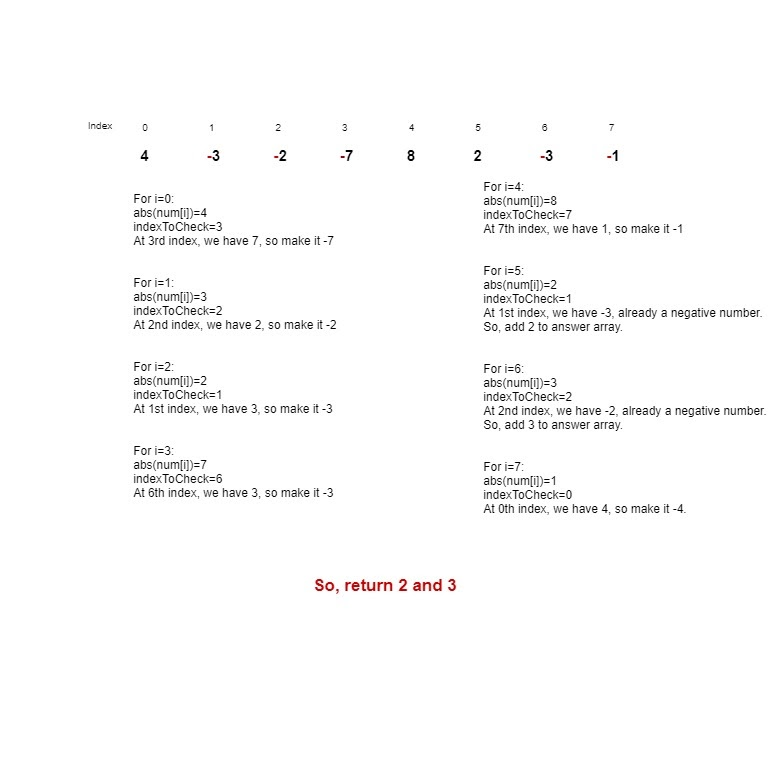# Find All Duplicates in an Array

## Introduction

In this blog, we will discuss an array problem that has been asked frequently in Interviews.

The problem is to Find All Duplicates in an Array.

We are given an array of N elements, and each integer appears once or twice. We have to return an array of all the integers that appears twice.

Example: nums = [4,3,2,7,8,2,3,1]

Here, 2 and 3 appear twice.

## Naive Approach

The naive approach to Find All Duplicates in an Array will be to take each element and to find whether any second occurrence of that element is present in the entire array or not. If any second occurrence is present, then we need to display it in the answer array. But this approach would take O(n²) time.

To have an optimised approach, we can also do this by using a HashSet.

This would reduce our Time Complexity and space complexity to O(n).

Declare a HashSet

If an element is not present in the HashSet on traversing the array, add the element to HashSet.

If that element is present in the HashSet, add the element to the result array.

Return the result array.

## Optimized Approach

As the condition to us is 1 <= nums[i] <= n, this means that number present in the array will not be greater than the length of the array so that we can reduce our space complexity to O(1).

get the index; the element corresponds to

Flip the number to negative

If the number is already negative, we encounter it twice, so store it in the answer array.

Return answer. i.e.:All Duplicates in an ArraySo in the most optimal approach, the time complexity for this problem will reduce to O(n) and space complexity to O(1).

Till now, I assume you must have got the basic idea of what has been asked in the problem statement. So, I strongly recommend you first give it a try.

Find All Duplicates In An Array

If you were not able to solve it, don’t be sad. It’s a part of the learning process.

Please have a look at the algorithm, and then again, you must give it a try.

### PseudoCode

Algorithm

___________________________________________________________________

procedure findDuplicates( ):

___________________________________________________________________

1.   Declare a vector to store the answer.

2.   Run a for loop from 0 till the size of nums.

3.   index=abs(nums[i])-1

4.   if(nums[index]<0)

5.   Store the number in the answer array.

6.   Else nums[index]=nums[index]*-1;

end procedure

___________________________________________________________________

### CODE IN C++

``````#include <iostream>
#include <bits/stdc++.h>
using namespace std;
int main() {
int n;
cin>>n;
int nums[n];
for(int i=0; i<n; i++){
cin>>nums[i];
}
vector<int> resultSet;
for(int i=0; i<n; i++){
//get the index, the element corresponds to
int index=abs(nums[i])-1;
//if present number is already negative, it means we are encountering it twice, so store in answer array.
if(nums[index]<0){
resultSet.push_back(index+1);
}
//Flip the number to negative
nums[index]=nums[index]*-1;
}
for(int i=0; i<resultSet.size(); i++){
cout<<resultSet[i]<<" ";
}
}``````

Output

``````Sample Input:
8
[4,3,2,7,8,2,3,1]
Sample Output:
[2,3]``````

#### Complexity Analysis

Time Complexity: O(n).

Analysing Time Complexity:

Since loop runs only once.

∴n.

Space complexity: O(1) since no extra variable is used.

1. How to approach a problem like this?
Answer) First, understand the problem. The best way is to use diagrams. Then write your algorithm. Then start with the Brute Force method. Lastly, try to optimise your code.
2. What is HashSet?
3. What is the difference between Array and Vector?
Answer) The array is static in size, whereas the vector is dynamic in size.

## Key Takeaways

This article taught us how to Find All Duplicates in an Array by approaching the problem using a hash set and then without extra space. We discussed its implementation using illustrations, pseudocode, and then proper code.

We hope you could take away critical techniques like analyzing problems by walking over the execution of the examples and finding out the recursive pattern followed in most binary search tree problems.
Now, we recommend you practice problem sets based on arrays to master your fundamentals. You can get a wide range of questions similar to this on CodeStudio

It's not the end. Must solve the problem of similar types.

Happy Coding.